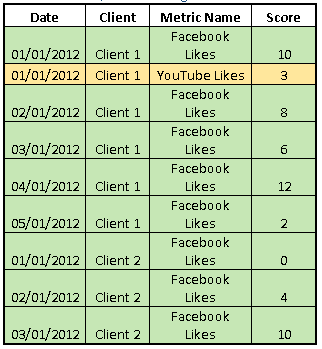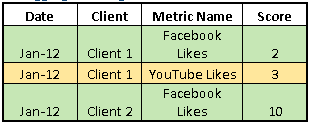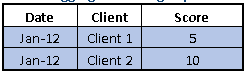# SSAS LastNonEmpty Aggregation Function

18 Aug
August 18, 2012

For some strange reason I can’t seem to find a lot of literature online about the basic behavior of the LastNonEmpty SSAS function, which is currently only available in an Enterprise Edition of Analysis Service (for some really strange reason, since you can emulate the behavior in other SSAS versions relatively painlessly), so here is a nice wee post that goes through the syntax of the LastNonEmpty function, how to override and customize it’s default behavior, what performance improvement techniques you can apply, and some of the quirks you might experience when playing around with the LastNonEmpty Analysis Service function.

## SSAS LastNonEmpty Aggregation Function Behavior

The LastNonEmpty function is a semi-additive function, and requires a dimension of type Time in order to be applicable (this can be set in the Dimension Properties), this is because it behaves differently when a measure is aggregating along the Time dimension, than it would when aggregating that same measure along any other (non-Time) dimension.

• LastNonEmpty Behavior along the Time Dimension: The last (earliest) existing value along the time segment is returned, so if you are aggregating monthly, then the value on the last day of the month that has a value is returned.
• LastNonEmpty Behavior along Non-Time Dimensions: The SUM aggregation function is applied, and the value returned is the SUM of the measure along all aggregated (non-Time typed) dimensions.

So lets take an example, the table below shows a simplified example that I’ve been working with recently. You can see there are 2 non-Time dimensions (Client and Metric Name), and 1 Time dimension (Date), in the table below it can be seen that Time is on a granularity level of Date, and the Score measure is aggregated using the LastNonEmpty SSAS function.Now if we aggregate the table above along the Time dimension, rolling up data to the Month per Year level, the Score will aggregate in the following manner:And now if we aggregate the table using the Metric Name dimension (by taking this dimension out), then the LastNonEmpty function will apply the SUM aggregation.## Overriding the LastNonEmpty Aggregation Behavior

Sometimes you might want to override the default behavior of the LastNonEmpty function, for example assume that you have a measure that you would like to aggregate using MAX rather than the default SUM you get with the LastNonEmpty function, you can do this by actually implementing the underlying LastNonEmpty function behavior using fundamental SSAS function, then customizing the behavior according to the requirement, for example if I want to override the SUM aggregation with MAX instead, I could apply the MAX aggregation on the measure itself, then create a Calculated Member using the following statement:

SCOPE([Measures].[MAX LastNonEmpty Measure]);
THIS = TAIL ( NONEMPTY ( { EXISTING [TIME].[DATE].[DATE].Members } * [Measures].[MAX Measure])
,1).ITEM(0);
END SCOPE;

The above could also be used to emulate the LastNonEmpty function in a non-Enterprise edition of Analysis Service, although apparently this will perform slower than simply using LastNonEmpty.

## Improve Performance of LastNonEmpty SSAS Function

Because the LastNonEmpty function tries to grab the last value of along the Time dimension that is non empty, it tends to behave much slower than the LastChild function, which does not discriminate whether the value is empty or not, and simply returns the last child along the Time dimension. So if you are noticing performance issues with the LastNonEmpty function then you could potentially switch to using the LastChild function, while implementing the Non-Empty behavior in the underlying fact table, possibly while ETL-ing onto that table.

Essentially all you would need to do is create a new column on your fact table that duplicates the Measure you are trying to implement the LastNonEmpty function on, but instead of having empty values in your column, you could fill in the empty gaps with the last available value (along the Time dimension) in your ETL process, then all you would need to do is call the LastChild function which will now also implement the Non-Empty behavior.

Ofcourse this does mean that you will need to change your underlying fact table, which could be troublesome, so if you would like to improve the LastNonEmpty Analysis Service function performance without changing schema, Chris Webb (SSAS Guru) covers a handy new approach to LastNonEmpty.

## LastNonEmpty Function Pitfalls

This function comes with a caveats with regard to Totals and Balances, in which it behaves in a less than expected manner, I won’t cover this here, but Paul te Braak has an awesome post that goes through the behavior of the Totals aggregation on a LastNonEmpty Measure.

SSAS LastNonEmpty Aggregation Function rated 4 out of 5 by 1 readers

SSAS LastNonEmpty Aggregation Function , 4.0 out of 5 based on 1 ratings
* * * * ½ 2 votes
1.